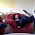## Thursday, 1 November 2018

### How to match "A B C" where A+B=C: The Beast Reborn

A while ago, I created a regex to verify addition of two non-negative integers, and unleashed the unholy mess onto reddit. Reactions were fierce, heads exploded, etc. and so I immediately took to demystifying it by introducing line breaks, comments, and structured formatting. This follow-up was so effective that many commenters were lulled to a state of boredom by the result, underwhelmed by its simplicity. The loudest voices expressed dissatisfaction with the robustness of the solution, complaining about the lack of negative number support and, worse yet, the stark absence of decimal support.

Slowly, I came to realize that these people were right. These features needed to be implemented; the regex simply wasn’t long and complicated enough. So I returned to the drawing board, puked on it several times, and ended up with the following:

# A slow start
^
# Immediately step on the gas
# Parse expression as before, but with some extra pizzazz
(?=[-+]?(?:0\B)*+(\d*?)((?:(?=\d+(?:\.\d+)?\ [-+]?(?:0\B)*+(\d*?)(\d(?(4)\4))(?:\.\d+)?\ [-+]?(?:0\B)*+(\d*?)(\d(?(6)\6))(?:\.\d+)?$)\d)++)\b) (?: # 0th case: handle 0 + 0 = 0 with any sign orientation ^(?:[-+]?0+(?:\.0+)?(?:\ |$)){3}\b$| # 1st case: handle "A B C" and "-A -B -C", ie. check A+B=C (?=-\S*\ -\S*\ -|(?!.*-)) [-+]?(?:0\B)*+ # Check leading excess digits (?= (?(?!\1\.?(?!(?!(?&a)))) # No carrying, match one excess part to the other (?=\S+\ \S+\ [-+]?0*\1\3\6(?:\.\d+)?$)
|

# Carrying, match one excess part to 1 + the other
(?(?!.*+\3)\S+\ [-+]?(?:0\B)*)
(?=\d*?(\2|\4)\b(.*?\ [-+]?(?:0\B)*)\S+$) # Check for carrying with all 9s (?(?=9*\7\b) # If so, match '9's to '0's and check for a leading '1' (?:9(?=\d*?\8(\g{-1}?+0)))*? \7\8\g{-1}?+\6\b | # Otherwise, match equivalent pairs of digits until they differ by 1 # Then pair any extra '0's with '9's (?:(\d)(?=\d*\8(\g{-1}?+\g{-2})))*+ (?=\d(\d*\8\g{-2}?+)) (?=0\g{-1}1|1\g{-1}2|2\g{-1}3|3\g{-1}4|4\g{-1}5|5\g{-1}6|6\g{-1}7|7\g{-1}8|8\g{-1}9) \d (?:9(?=\d*\8\g{-2}?+\d(\g{-1}?+0)))*? \7\8\g{-3}?+\d\g{-1}?+\6\b ) ) ) \1 # Check rest of digits (?: # Identify next group of digits to examine (?=\.?(\d)\S*\ [-+]?(?:0\B)*+\3((\g{-2}?+\.?)\d)\S*\ [-+]?(?:0\B)*+\5((\g{-2}?+\.?)\d)) \.?(*SKIP) # Check for carrying and match digits accordingly (?(?=(?! \14\.? (?<a> # Match pairs of digits that total 9 (?: (?=\d\.?\d(?<b>\S*?\ [-+]?(?:0\B)*\3\15?+)((\g{-2}?+\.?)\d)) (?=\d(\S*?\g{-4}\g{-2})) (?=0\g{-1}9|1\g{-1}8|2\g{-1}7|3\g{-1}6|4\g{-1}5|5\g{-1}4|6\g{-1}3|7\g{-1}2|8\g{-1}1|9\g{-1}0) \d\.?(?=\S*?(\g{-5}\g{-4})) )*+ # Match a pair of digits that totals > 9 (?=\d(\g{-2}\.?|(?&b)\.?)) (?=[5-9]\g{-1}[5-9]|1\g{-1}9|2\g{-1}|3\g{-1}[7-9]|4\g{-1}[6-9]|6\g{-1}4|7\g{-1}|8\g{-1}[2-4]|9\g{-1}[1-4]) ){0} # Backreferences were erroneously being set that carried over to subsequent iterations, # necessitating this subroutine call # PCRE really wanted me to fail, apparently (?&a) )) # No carrying, match pairs of digits and their sums # My goodness it's beautiful (?=\d(\S*\ [-+]?(?:0\B)*\3\16)\d(\S*\ [-+]?(?:0\B)*\5\18)) (?= 1\g{-2}(?:1\g{-1}2|2\g{-1}3|3\g{-1}4|4\g{-1}5|5\g{-1}6|6\g{-1}7|7\g{-1}8|8\g{-1}9|9\g{-1}0) |2\g{-2}(?:1\g{-1}3|2\g{-1}4|3\g{-1}5|4\g{-1}6|5\g{-1}7|6\g{-1}8|7\g{-1}9|8\g{-1}0|9\g{-1}1) |3\g{-2}(?:1\g{-1}4|2\g{-1}5|3\g{-1}6|4\g{-1}7|5\g{-1}8|6\g{-1}9|7\g{-1}0|8\g{-1}1|9\g{-1}2) |4\g{-2}(?:1\g{-1}5|2\g{-1}6|3\g{-1}7|4\g{-1}8|5\g{-1}9|6\g{-1}0|7\g{-1}1|8\g{-1}2|9\g{-1}3) |5\g{-2}(?:1\g{-1}6|2\g{-1}7|3\g{-1}8|4\g{-1}9|5\g{-1}0|6\g{-1}1|7\g{-1}2|8\g{-1}3|9\g{-1}4) |6\g{-2}(?:1\g{-1}7|2\g{-1}8|3\g{-1}9|4\g{-1}0|5\g{-1}1|6\g{-1}2|7\g{-1}3|8\g{-1}4|9\g{-1}5) |7\g{-2}(?:1\g{-1}8|2\g{-1}9|3\g{-1}0|4\g{-1}1|5\g{-1}2|6\g{-1}3|7\g{-1}4|8\g{-1}5|9\g{-1}6) |8\g{-2}(?:1\g{-1}9|2\g{-1}0|3\g{-1}1|4\g{-1}2|5\g{-1}3|6\g{-1}4|7\g{-1}5|8\g{-1}6|9\g{-1}7) |9\g{-2}(?:1\g{-1}0|2\g{-1}1|3\g{-1}2|4\g{-1}3|5\g{-1}4|6\g{-1}5|7\g{-1}6|8\g{-1}7|9\g{-1}8) |0\g{-2}(\d)\g{-2}\g{-1} |(\d)\g{-4}0\g{-3}\g{-1} ) | # Carrying, match pairs of digits and their sums + 1 (?=\d((?-5))\d((?-5))) (?= 1\g{-2}(?:0\g{-1}2|1\g{-1}3|2\g{-1}4|3\g{-1}5|4\g{-1}6|5\g{-1}7|6\g{-1}8|7\g{-1}9|8\g{-1}0|9\g{-1}1) |2\g{-2}(?:0\g{-1}3|1\g{-1}4|2\g{-1}5|3\g{-1}6|4\g{-1}7|5\g{-1}8|6\g{-1}9|7\g{-1}0|8\g{-1}1|9\g{-1}2) |3\g{-2}(?:0\g{-1}4|1\g{-1}5|2\g{-1}6|3\g{-1}7|4\g{-1}8|5\g{-1}9|6\g{-1}0|7\g{-1}1|8\g{-1}2|9\g{-1}3) |4\g{-2}(?:0\g{-1}5|1\g{-1}6|2\g{-1}7|3\g{-1}8|4\g{-1}9|5\g{-1}0|6\g{-1}1|7\g{-1}2|8\g{-1}3|9\g{-1}4) |5\g{-2}(?:0\g{-1}6|1\g{-1}7|2\g{-1}8|3\g{-1}9|4\g{-1}0|5\g{-1}1|6\g{-1}2|7\g{-1}3|8\g{-1}4|9\g{-1}5) |6\g{-2}(?:0\g{-1}7|1\g{-1}8|2\g{-1}9|3\g{-1}0|4\g{-1}1|5\g{-1}2|6\g{-1}3|7\g{-1}4|8\g{-1}5|9\g{-1}6) |7\g{-2}(?:0\g{-1}8|1\g{-1}9|2\g{-1}0|3\g{-1}1|4\g{-1}2|5\g{-1}3|6\g{-1}4|7\g{-1}5|8\g{-1}6|9\g{-1}7) |8\g{-2}(?:0\g{-1}9|1\g{-1}0|2\g{-1}1|3\g{-1}2|4\g{-1}3|5\g{-1}4|6\g{-1}5|7\g{-1}6|8\g{-1}7|9\g{-1}8) |9\g{-2}(?:0\g{-1}0|1\g{-1}1|2\g{-1}2|3\g{-1}3|4\g{-1}4|5\g{-1}5|6\g{-1}6|7\g{-1}7|8\g{-1}8|9\g{-1}9) |0\g{-2}(?:0\g{-1}1|1\g{-1}2|2\g{-1}3|3\g{-1}4|4\g{-1}5|5\g{-1}6|6\g{-1}7|7\g{-1}8|8\g{-1}9|9\g{-1}0) ) ) \d )++ # Paths to success (?: # A, B and C contain no more significant digits (?:\.0+)?0*\ [-+]?0*\3\15(?:\.0+)?0*\ [-+]?0*\5\17(?:\.0+)?0*$
|
# A contains no more significant digits, match B's excess to C's
\ [-+]?0*\3\15(\.?\d*?)0*\ [-+]?0*\5\17\g{-1}(?:\.0+)?0*$| # B contains no more significant digits, match A's excess to C's (\.?\d*?)0*\ [-+]?0*\3\15\ [-+]?0*\5\17\g{-1}(?:\.0+)?0*$
|
# C contains no more significant digits but A and B both do
# Match pairs of digits that total 9 until one that totals 10 is found
\.?
(?:
(?=\d\d((?&b))((\g{-2}?+\.?)\d))
(?=\d(\S*?\g{-4}\g{-2}))
(?=0\g{-1}9|1\g{-1}8|2\g{-1}7|3\g{-1}6|4\g{-1}5|5\g{-1}4|6\g{-1}3|7\g{-1}2|8\g{-1}1|9\g{-1}0)
\d
(?=\S*?(\g{-5}(\g{-5})))
)*+
# Match a pair that totals 10 then validate
(?=\d(\g{-3}|(?&b)\.?))
(?=1\g{-1}9|2\g{-1}8|3\g{-1}7|4\g{-1}6|5\g{-1}5|6\g{-1}4|7\g{-1}3|8\g{-1}2|9\g{-1}1)
\d0*\ [-+]?0*\3\15\g{-2}?+\.?\d0*\ [-+]?0*\5\17\.?0*$) | # Case 2: handle "-A B C" and "A -B -C", ie. check A+C=B (?=-(?!.*-)|[^-]\S*\ -\S*\ -) [-+]?(?:0\B)*+ # Check leading excess digits (?= (?(?!\1\.?(?!(?!(?&c)))) # No carrying, match one excess part to the other (?=\S+\ [-+]?0*\1\5\4\b) | # Carrying, match one excess part to 1 + the other # There are two paths here depending on whether |A| < |C| or |C| <= |A| (?| # |A| < |C| (?!.++\5) \S+\ [-+]?(?:0\B)*+(?=\S*(\ [-+]?(?:0\B)*+)()) # Check for carrying with all '9's (?(?=\S*\41*\6\b) # If so, match '9's to '0's and check for a leading '1' 1(?:0(?=\S*\41(\g{-1}?+9)))*? \4\b\S*\41\g{-1}?+\6\b | # Otherwise, match equivalent pairs of digits until they differ by 1 # Then pair any extra '0's with '9's (?:(\d)(?=\S*\41(\g{-1}?+\g{-2})))*+ (?=\d(\S*\41\g{-2}?+))(?=1\g{-1}0|2\g{-1}1|3\g{-1}2|4\g{-1}3|5\g{-1}4|6\g{-1}5|7\g{-1}6|8\g{-1}7|9\g{-1}8) \d (?:0(?=\S*\41\g{-2}?+\d(\g{-1}?+9)))*? \4\b\S*\41\g{-3}?+\d\g{-1}?+\6\b ) | # |C| <= |A|, basically the same comments as above (?=.*+\5) (?=\d*?(\2)\b(\S*\ [-+]?(?:0\B)*+)) (?(?=9*\41\b) (?:9(?=\d*?\42(\g{-1}?+0)))*? \41\42\g{-1}?+\4\b | (?:(\d)(?=\d*\42(\g{-1}?+\g{-2})))*+ (?=\d(\d*\42\g{-2}?+)) (?=0\g{-1}1|1\g{-1}2|2\g{-1}3|3\g{-1}4|4\g{-1}5|5\g{-1}6|6\g{-1}7|7\g{-1}8|8\g{-1}9) \d (?:9(?=\S*\42\g{-2}?+\d(\g{-1}?+0)))*? \41\42\g{-3}?+\d\g{-1}?+\4\b ) ) ) ) \1 # Check rest of digits (?: # Identify next group of digits to examine (?=\.?(\d)\S*\ [-+]?(?:0\B)*+\3((\g{-2}?+\.?)\d)\S*\ [-+]?(?:0\B)*+\5((\g{-2}?+\.?)\d)) \.?(*SKIP) # Check for carrying and match digits accordingly (?(?=(?! \48\.? (?<c> # Match pairs of digits that total 9 (?: (?=\d\.?\d(?<d>\S*?\ \S+\ [-+]?(?:0\B)*\5\51?+)((\g{-2}?+\.?)\d)) (?=\d(\S*?\g{-4}\g{-2})) (?=0\g{-1}9|1\g{-1}8|2\g{-1}7|3\g{-1}6|4\g{-1}5|5\g{-1}4|6\g{-1}3|7\g{-1}2|8\g{-1}1|9\g{-1}0) \d\.?(?=\S*?(\g{-5}\g{-4})) )*+ # Match a pair of digits that totals > 9 (?=\d(\g{-2}\.?|(?&d)\.?)) (?=[5-9]\g{-1}[5-9]|1\g{-1}9|2\g{-1}|3\g{-1}[7-9]|4\g{-1}[6-9]|6\g{-1}4|7\g{-1}|8\g{-1}[2-4]|9\g{-1}[1-4]) ){0} # Backreferences were erroneously being set that carried over to subsequent iterations, # necessitating this silly subroutine call (?&c) )) # No carrying, match pairs of digits and their sums (?=\d(\S*\ [-+]?(?:0\B)*\3\50)\d(\S*\ [-+]?(?:0\B)*\5\52)) (?= 1\g{-2}(?:2\g{-1}1|3\g{-1}2|4\g{-1}3|5\g{-1}4|6\g{-1}5|7\g{-1}6|8\g{-1}7|9\g{-1}8|0\g{-1}9) |2\g{-2}(?:3\g{-1}1|4\g{-1}2|5\g{-1}3|6\g{-1}4|7\g{-1}5|8\g{-1}6|9\g{-1}7|0\g{-1}8|1\g{-1}9) |3\g{-2}(?:4\g{-1}1|5\g{-1}2|6\g{-1}3|7\g{-1}4|8\g{-1}5|9\g{-1}6|0\g{-1}7|1\g{-1}8|2\g{-1}9) |4\g{-2}(?:5\g{-1}1|6\g{-1}2|7\g{-1}3|8\g{-1}4|9\g{-1}5|0\g{-1}6|1\g{-1}7|2\g{-1}8|3\g{-1}9) |5\g{-2}(?:6\g{-1}1|7\g{-1}2|8\g{-1}3|9\g{-1}4|0\g{-1}5|1\g{-1}6|2\g{-1}7|3\g{-1}8|4\g{-1}9) |6\g{-2}(?:7\g{-1}1|8\g{-1}2|9\g{-1}3|0\g{-1}4|1\g{-1}5|2\g{-1}6|3\g{-1}7|4\g{-1}8|5\g{-1}9) |7\g{-2}(?:8\g{-1}1|9\g{-1}2|0\g{-1}3|1\g{-1}4|2\g{-1}5|3\g{-1}6|4\g{-1}7|5\g{-1}8|6\g{-1}9) |8\g{-2}(?:9\g{-1}1|0\g{-1}2|1\g{-1}3|2\g{-1}4|3\g{-1}5|4\g{-1}6|5\g{-1}7|6\g{-1}8|7\g{-1}9) |9\g{-2}(?:0\g{-1}1|1\g{-1}2|2\g{-1}3|3\g{-1}4|4\g{-1}5|5\g{-1}6|6\g{-1}7|7\g{-1}8|8\g{-1}9) |0\g{-2}(\d)\g{-2}\g{-1} |(\d)\g{-4}\g{-1}\g{-3}0 ) | # Carrying, match pairs of digits and their sums + 1 (?=\d((?-5))\d((?-5))) (?= 1\g{-2}(?:2\g{-1}0|3\g{-1}1|4\g{-1}2|5\g{-1}3|6\g{-1}4|7\g{-1}5|8\g{-1}6|9\g{-1}7|0\g{-1}8|1\g{-1}9) |2\g{-2}(?:3\g{-1}0|4\g{-1}1|5\g{-1}2|6\g{-1}3|7\g{-1}4|8\g{-1}5|9\g{-1}6|0\g{-1}7|1\g{-1}8|2\g{-1}9) |3\g{-2}(?:4\g{-1}0|5\g{-1}1|6\g{-1}2|7\g{-1}3|8\g{-1}4|9\g{-1}5|0\g{-1}6|1\g{-1}7|2\g{-1}8|3\g{-1}9) |4\g{-2}(?:5\g{-1}0|6\g{-1}1|7\g{-1}2|8\g{-1}3|9\g{-1}4|0\g{-1}5|1\g{-1}6|2\g{-1}7|3\g{-1}8|4\g{-1}9) |5\g{-2}(?:6\g{-1}0|7\g{-1}1|8\g{-1}2|9\g{-1}3|0\g{-1}4|1\g{-1}5|2\g{-1}6|3\g{-1}7|4\g{-1}8|5\g{-1}9) |6\g{-2}(?:7\g{-1}0|8\g{-1}1|9\g{-1}2|0\g{-1}3|1\g{-1}4|2\g{-1}5|3\g{-1}6|4\g{-1}7|5\g{-1}8|6\g{-1}9) |7\g{-2}(?:8\g{-1}0|9\g{-1}1|0\g{-1}2|1\g{-1}3|2\g{-1}4|3\g{-1}5|4\g{-1}6|5\g{-1}7|6\g{-1}8|7\g{-1}9) |8\g{-2}(?:9\g{-1}0|0\g{-1}1|1\g{-1}2|2\g{-1}3|3\g{-1}4|4\g{-1}5|5\g{-1}6|6\g{-1}7|7\g{-1}8|8\g{-1}9) |9\g{-2}(?:0\g{-1}0|1\g{-1}1|2\g{-1}2|3\g{-1}3|4\g{-1}4|5\g{-1}5|6\g{-1}6|7\g{-1}7|8\g{-1}8|9\g{-1}9) |0\g{-2}(?:1\g{-1}0|2\g{-1}1|3\g{-1}2|4\g{-1}3|5\g{-1}4|6\g{-1}5|7\g{-1}6|8\g{-1}7|9\g{-1}8|0\g{-1}9) ) ) \d )++ # Paths to success (?: # A, B, and C contain no more significant digits (?:\.0+)?0*\ [-+]?0*\3\49(?:\.0+)?0*\ [-+]?0*\5\51(?:\.0+)?0*$

|

# A contains no more significant digits, match B's excess to C's
\ [-+]?0*\3\49(\.?\d*?)0*\ [-+]?0*\5\51\g{-1}(?:\.0+)?0*$| # C contains no more significant digits, match A's excess to B's (\.?\d*?)0*\ [-+]?0*\3\49\g{-1}(?:\.0+)?0*\ [-+]?0*\5\51$

|

# B contains no more significant digits but A and B both do
# Match pairs of digits that total 9 until one that totals 10 is found
\.?
(?:
(?=\d\d((?&d))((\g{-2}?+\.?)\d))
(?=\d(\S*?\g{-4}\g{-2}))
(?=0\g{-1}9|1\g{-1}8|2\g{-1}7|3\g{-1}6|4\g{-1}5|5\g{-1}4|6\g{-1}3|7\g{-1}2|8\g{-1}1|9\g{-1}0)
\d
(?=\S*?(\g{-5}(\g{-5})))
)*+
# Match a pair that totals 10 then validate
(?=\d(\g{-3}|(?&d)\.?))
(?=1\g{-1}9|2\g{-1}8|3\g{-1}7|4\g{-1}6|5\g{-1}5|6\g{-1}4|7\g{-1}3|8\g{-1}2|9\g{-1}1)
\d0*\ [-+]?0*\3\49\.?0*\ [-+]?0*\5\51\g{-2}?+\.?\d0*$) | # And finally, handle "A -B C" and "-A B -C", ie. check B+C=A (?=[^-]\S*\ -\S*\ [^-]\S*|-\S*\ [^-]\S*\ -) [-+]?(?:0\B)*+ # Check leading excess digits (?= (?(?!\S+\ [-+]?(?:0\B)*+\3\.?(?!(?!(?&e)))) # No carrying, match one excess part to the other (?=\3\5\2\b) | # Carrying, match one excess part to 1 + the other (?=\d*(\S*\ (?(?=.*+\3)\S+\ )[-+]?(?:0\B)*+)\d*?(\4|\6)\b) # Check for carrying with a leading '1' and all '0's (?(?=10*\2\b) # If so, match '0's to '9's 1(?:0(?=\d*\75(\g{-1}?+9)))*? \2\b\75\g{-1}?+\76\b | # Otherwise, match equivalent pairs of digits until they differ by 1 # Then pair any extra '0's with '9's (?:(\d)(?=\d*\75(\g{-1}?+\g{-2})))*+ (?=\d(\d*\75\g{-2}?+)) (?=1\g{-1}0|2\g{-1}1|3\g{-1}2|4\g{-1}3|5\g{-1}4|6\g{-1}5|7\g{-1}6|8\g{-1}7|9\g{-1}8) \d (?:0(?=\d*\75\g{-2}?+\d(\g{-1}?+9)))*? \2\b\75\g{-3}?+\d\g{-1}?+\76\b ) ) ) \1 # Check rest of digits (?: # Identify next group of digits to examine (?=\.?(\d)\S*\ [-+]?(?:0\B)*+\3((\g{-2}?+\.?)\d)\S*\ [-+]?(?:0\B)*+\5((\g{-2}?+\.?)\d)) \.?(*SKIP) # Check for carrying and match digits accordingly (?(?=(?! \S*\ [-+]?(?:0\B)*+\3\83\.? (?<e> # Match pairs of digits that total 9 (?: (?=\d\.?\d(?<f>\S*?\ [-+]?(?:0\B)*\5\85?+)((\g{-2}?+\.?)\d)) (?=\d(\S*?\g{-4}\g{-2})) (?=0\g{-1}9|1\g{-1}8|2\g{-1}7|3\g{-1}6|4\g{-1}5|5\g{-1}4|6\g{-1}3|7\g{-1}2|8\g{-1}1|9\g{-1}0) \d\.? (?=\S*?(\g{-5}\g{-4})) )*+ # Match a pair of digits that totals > 9 (?=\d(\g{-2}\.?|(?&f)\.?)) (?=[5-9]\g{-1}[5-9]|1\g{-1}9|2\g{-1}|3\g{-1}[7-9]|4\g{-1}[6-9]|6\g{-1}4|7\g{-1}|8\g{-1}[2-4]|9\g{-1}[1-4]) ){0} # Backreferences were erroneously being set that carried over to subsequent iterations, # necessitating this preposterous subroutine call (?&e) )) # No carrying, match pairs of digits and their sums (?=\d(\S*\ [-+]?(?:0\B)*\3\84)\d(\S*\ [-+]?(?:0\B)*\5\86)) (?= (?:2\g{-2}1|3\g{-2}2|4\g{-2}3|5\g{-2}4|6\g{-2}5|7\g{-2}6|8\g{-2}7|9\g{-2}8|0\g{-2}9)\g{-1}1 |(?:3\g{-2}1|4\g{-2}2|5\g{-2}3|6\g{-2}4|7\g{-2}5|8\g{-2}6|9\g{-2}7|0\g{-2}8|1\g{-2}9)\g{-1}2 |(?:4\g{-2}1|5\g{-2}2|6\g{-2}3|7\g{-2}4|8\g{-2}5|9\g{-2}6|0\g{-2}7|1\g{-2}8|2\g{-2}9)\g{-1}3 |(?:5\g{-2}1|6\g{-2}2|7\g{-2}3|8\g{-2}4|9\g{-2}5|0\g{-2}6|1\g{-2}7|2\g{-2}8|3\g{-2}9)\g{-1}4 |(?:6\g{-2}1|7\g{-2}2|8\g{-2}3|9\g{-2}4|0\g{-2}5|1\g{-2}6|2\g{-2}7|3\g{-2}8|4\g{-2}9)\g{-1}5 |(?:7\g{-2}1|8\g{-2}2|9\g{-2}3|0\g{-2}4|1\g{-2}5|2\g{-2}6|3\g{-2}7|4\g{-2}8|5\g{-2}9)\g{-1}6 |(?:8\g{-2}1|9\g{-2}2|0\g{-2}3|1\g{-2}4|2\g{-2}5|3\g{-2}6|4\g{-2}7|5\g{-2}8|6\g{-2}9)\g{-1}7 |(?:9\g{-2}1|0\g{-2}2|1\g{-2}3|2\g{-2}4|3\g{-2}5|4\g{-2}6|5\g{-2}7|6\g{-2}8|7\g{-2}9)\g{-1}8 |(?:0\g{-2}1|1\g{-2}2|2\g{-2}3|3\g{-2}4|4\g{-2}5|5\g{-2}6|6\g{-2}7|7\g{-2}8|8\g{-2}9)\g{-1}9 |(\d)\g{-3}0\g{-2}\g{-1} |(\d)\g{-4}\g{-1}\g{-3}0 ) | # Carrying, match pairs of digits and their sums + 1 (?=\d((?-5))\d((?-5))) (?= (?:2\g{-2}0|3\g{-2}1|4\g{-2}2|5\g{-2}3|6\g{-2}4|7\g{-2}5|8\g{-2}6|9\g{-2}7|0\g{-2}8|1\g{-2}9)\g{-1}1 |(?:3\g{-2}0|4\g{-2}1|5\g{-2}2|6\g{-2}3|7\g{-2}4|8\g{-2}5|9\g{-2}6|0\g{-2}7|1\g{-2}8|2\g{-2}9)\g{-1}2 |(?:4\g{-2}0|5\g{-2}1|6\g{-2}2|7\g{-2}3|8\g{-2}4|9\g{-2}5|0\g{-2}6|1\g{-2}7|2\g{-2}8|3\g{-2}9)\g{-1}3 |(?:5\g{-2}0|6\g{-2}1|7\g{-2}2|8\g{-2}3|9\g{-2}4|0\g{-2}5|1\g{-2}6|2\g{-2}7|3\g{-2}8|4\g{-2}9)\g{-1}4 |(?:6\g{-2}0|7\g{-2}1|8\g{-2}2|9\g{-2}3|0\g{-2}4|1\g{-2}5|2\g{-2}6|3\g{-2}7|4\g{-2}8|5\g{-2}9)\g{-1}5 |(?:7\g{-2}0|8\g{-2}1|9\g{-2}2|0\g{-2}3|1\g{-2}4|2\g{-2}5|3\g{-2}6|4\g{-2}7|5\g{-2}8|6\g{-2}9)\g{-1}6 |(?:8\g{-2}0|9\g{-2}1|0\g{-2}2|1\g{-2}3|2\g{-2}4|3\g{-2}5|4\g{-2}6|5\g{-2}7|6\g{-2}8|7\g{-2}9)\g{-1}7 |(?:9\g{-2}0|0\g{-2}1|1\g{-2}2|2\g{-2}3|3\g{-2}4|4\g{-2}5|5\g{-2}6|6\g{-2}7|7\g{-2}8|8\g{-2}9)\g{-1}8 |(?:0\g{-2}0|1\g{-2}1|2\g{-2}2|3\g{-2}3|4\g{-2}4|5\g{-2}5|6\g{-2}6|7\g{-2}7|8\g{-2}8|9\g{-2}9)\g{-1}9 |(?:1\g{-2}0|2\g{-2}1|3\g{-2}2|4\g{-2}3|5\g{-2}4|6\g{-2}5|7\g{-2}6|8\g{-2}7|9\g{-2}8|0\g{-2}9)\g{-1}0 ) ) \d )++ # Paths to success (?: # A, B, and C contain no more significant digits (?:\.0+)?0*\ [-+]?0*\3\83(?:\.0+)?0*\ [-+]?0*\5\85(?:\.0+)?0*$
|
# C contains no more significant digits, match A's excess to B's
(\.?\d*?)0*\ [-+]?0*\3\83\g{-1}(?:\.0+)?0*\ [-+]?0*\5\85$| # B contains no more significant digits, match A's excess to C's (\.?\d*?)0*\ [-+]?0*\3\83\ [-+]?0*\5\85\g{-1}(?:\.0+)?0*$
|
# A contains no more significant digits but B and C both do
# Match pairs of digits that total 9 until one that totals 10 is found
\.?0*\ [-+]?(?:0\B)*+\3\83\.?
(?:
(?=\d\d((?&f))((\g{-2}?+\.?)\d))
(?=\d(\S*?\g{-4}\g{-2}))
(?=0\g{-1}9|1\g{-1}8|2\g{-1}7|3\g{-1}6|4\g{-1}5|5\g{-1}4|6\g{-1}3|7\g{-1}2|8\g{-1}1|9\g{-1}0)
\d
(?=\S*?(\g{-5}(\g{-5})))
)*+
# Match a pair that totals 10 then validate
(?=\d(\g{-3}|(?&f)\.?))
(?=1\g{-1}9|2\g{-1}8|3\g{-1}7|4\g{-1}6|5\g{-1}5|6\g{-1}4|7\g{-1}3|8\g{-1}2|9\g{-1}1)
\d0*\ [-+]?0*\5\85\g{-2}?+\.?\d0*\$
)
)


This was a fantastic personal challenge that really pushed me to consider how every conceivable arrangement of digits would be matched by each piece of the regex. When, say, test case #2,490,511 erroneously failed to match and the only hint given consists solely of the three numbers that caused the failure, it’s a little tricky to know where to begin debugging. This happened over and over, prompting revision after revision, until - finally - it stopped. When I could safely conclude that the regex was fully functional, that was a very satisfying “Eureka” moment indeed.

The general method is similar to the simple, positive-integer regex that I commented exhaustively last time, and so I didn't feel the need to add as many comments this time. The individual algorithms are almost the same, but the general method has of course been expanded: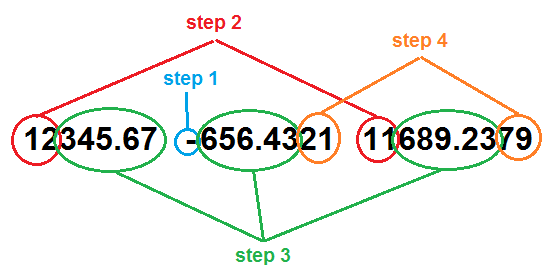Step 1: Examine the signs of A, B, and C to determine the order of matching. There are eight possible combinations of signs: two complementary arrangements for when each of A, B, and C appear as the highest value (ie. the sum of the addition), and two invalid arrangements (“-A –B C” and “A B –C”).

In the above example, an arrangement of “A –B C” calls for verifying that B + C = A, ie. 12345.67 = 656.4321 + 11689.2379.

Step 2: Compare the excess part of the total with the excess part of the longer of the two operands, similar to last time. They will either be equal or differ by exactly 1. Note that this comparison cannot spill over into the decimal portion.

In our example, we find the excess part of A, “12”, should be compared to the excess part of C, “11”. After pairing ‘3’ and ‘6’, which total 9, we find ‘4’ and ‘8’, which yield a sum greater than 9. And so we can rightly match “12” against “11” since they differ by 1.

Step 3: Compare the equal-length portions of A, B, and C. Like before, this is done by matching pairs of digits in the two operands to their sums in the total. However, unlike before, this matching may continue into the decimal portions of the 3 numbers.

In the example, ‘3’ matches with ‘6’ and ‘6’ because of carrying (6+6=12, then + 1 = 3), and then ‘4’ matches with ‘5’ and ‘8’, and so on until A runs out of digits.

Step 4: At this stage, if the addition is correct then the only digits that could possibly remain are decimals. In fact, these residual decimal digits would exist in exactly two of A, B, or C, and there would be the same number of digits left in both. If one of these two is the total, then our final check is basic: make sure the two residual parts are equivalent (for example, 1.2 + 1.2345 = 2.4345). Otherwise, we need to match pairs of digits that total 9 until the last pair, which should total 10.

So in our example, we are left with “21” in B and “79” in C. ‘2’ and ‘7’ total 9, and then ‘9’ and ‘1’ give us 10 as needed.

If you've made it to here without closing the page in disgust, and if things of this nature appeal to you, then I invite you to follow me on Twitter (@DrRegex) and/or subscribe to this blog to get regular updates. I do have more in store.

1.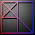So this is what programming with Satan is like.

1.#Truth #Preach

2.2.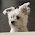This comment has been removed by the author.

3.Most impressive.

1.Thank you very much!

4.As someone mentioned in the reddit thread the following two cases give false positives...
100 100 201
100 100 210

1.Actually its any number from 201 and 210

2.Done - thank you :)

5.6.7.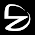8.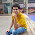9.